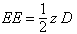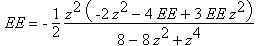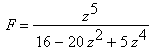Erich Prisner

# Systems of Recurrence Relations

Several sequences a0, a1, a2, ... ; b0, b1, b2, ... ; etc may be given by recurrence relations between different sequences. Here is an example (stemming from random walks) with six sequences: (note that the e-sequence is abbreviated by ee to distinguish from the number e).

 an=bn-1/2 bn=an+cn-1/2 cn=bn/2+dn-1/2 dn=cn/2+een-1/2 een=dn-1/2 fn=een-1/2

with initial conditions a0=1, b0=0, c0=0, d0=0, ee0=0, f0=0.We make use of the special character of the equations and use substitution method to get a formula for F(z): First we solve the first equation for A,, and substitute this into the second equation, solve this for B to get, substitute this into the third to get, and, and, and finally.

Take the following example:

Erich Prisner 2004